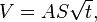# Capillary action speed formula?

Hi,

I was looking for a formula to describe capillary action speed in a medium.

On wikipedia (https://en.wikipedia.org/wiki/Capillary_action) I came across a formula which describes the cumulative volume of a liquid drawn by capillary action after time twhere V is cumulative volume, A is area, S is sorptivity and t is time.

If I reverse this to

t = (V/AS)2, is this the speed/time correlation I'm looking for, or is it just a relation with volume?

Thank you

Allison

•